## Orthogonal Vector

Two vectors are perpendicular (or orthogonal) to each other if and only if their inner product is zero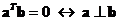. Vectors that perpendicular to each other are also called orthogonal vectors .

When the two vectors that perpendicular to each other also have unit length (i.e. their norm is one), then these vectors are called orthonormal vectors .

The interactive program below will help you to determine whether your input vectors are orthogonal or not. When you click random example button, the program will give you a lot of examples of both orthogonal vectors and not orthogonal vectors.

vector x vector y

## Properties

Some important properties of orthogonal & orthonormal vector are

• Two unit vectors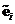and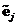are perpendicular to each other (orthogonal) if and only if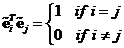.
• In 3-dimensional Euclidean space, there are 3 standard unit vectors that orthogonal to each other with special name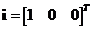,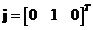and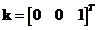. Figure below show the 3 standard orthogonal unit vectors.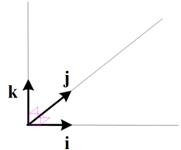• The dot products of the standard orthogonal unit vector:
• Dot product of the same standard unit vector is one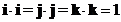• Dot product of the orthogonal standard unit vector is zero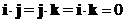• The cross product of the standard unit vectors:
• Cross product of the same standard unit vector is zero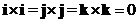• Cross product of the orthogonal standard unit vector form a cycle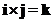;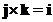;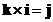;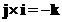;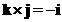;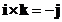;